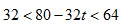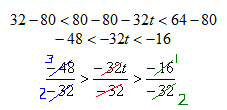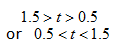Example 1

The velocity of an object fired directly upward is given by V = 80 - 32t, where t is in seconds. When will the velocity be between 32 and 64 feet per second?

Step 1. Set up the compound inequality.Step 2. Solve for t.(Reminder: since the inequality is divided through by a negative,
the inequality signs need to be reversed.)